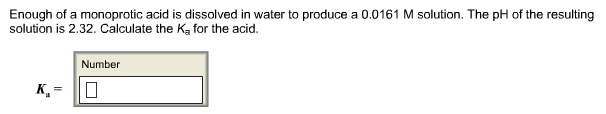# Problem: Enough of a monoprotic acid is dissolved in water to produce a 0.0161 M solution. The pH of the resulting solution is 2.32. Calculate the Ka for the acid. Ka =

###### FREE Expert Solution
84% (294 ratings)###### Problem Details

Enough of a monoprotic acid is dissolved in water to produce a 0.0161 M solution. The pH of the resulting solution is 2.32. Calculate the Ka for the acid.

Ka